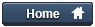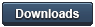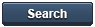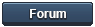### Gammon Forum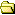Entire forum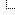Electronics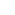Microprocessors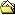ADC conversion on the Arduino (analogRead)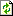Refresh page

Posted by Nick Gammon   Australia  (22,921 posts)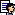bio   Forum Administrator
Date Fri 27 Feb 2015 08:24 AM (UTC)

Amended on Fri 05 Feb 2016 04:24 AM (UTC) by Nick Gammon

Message

Introduction

The Arduino has an ADC (Analog to Digital Converter) which is connected to various input pins on the board. In the case of the Uno they are labelled A0 to A5.

The basic usage is very simple:

``````
``````

The results are a number in the range 0 to 1023.

Using default settings, a return value of 0 would represent approximately 0V, and a return value of 1023 (the maximum) would represent approximately 5V. However more about the exact conversion details later.

This thread explores various things you can do with the ADC, including:

• Speeding it up
• Using different reference voltages
• Making it non-blocking
• Using an interrupt
• Reading other things such as the internal chip temperature
• Measuring resistances
• Measuring voltages higher than 5V
• Interpreting the results accurately

Timing

The ADC takes 13 ADC clock cycles to perform a conversion, except the first one after the ADC is enabled, at which time it takes 25 ADC cycles, while the circuitry is initialized.

You can choose various prescalers, from 2 to 128. This divides down the processor clock speed to give an ADC clock speed. You can do that by changing the ADCSRA register, like this:

``````

``````

The conversion time will be 13 times the clock period (or 25 for the first conversion) thus each one would be (assuming a 16 MHz clock):

``````
Prescaler
2    * 13 * 1/16E6  = 0.000001625 (  1.625 µs)
4    * 13 * 1/16E6  = 0.00000325  (  3.25  µs)
8    * 13 * 1/16E6  = 0.0000065   (  6.5   µs)
16    * 13 * 1/16E6  = 0.000013    ( 13     µs)
32    * 13 * 1/16E6  = 0.000026    ( 26     µs)
64    * 13 * 1/16E6  = 0.000052    ( 52     µs)
128    * 13 * 1/16E6  = 0.000104    (104     µs)
``````

Taking the inverse of the period we can work out the maximum theoretical conversion rate per second:

``````
Prescaler  Conversions/sec
2           615,385
4           307,692
8           153,846
16            76,923
32            38,462
64            19,231
128             9,615
``````

Of course, it won't ever get that good because there would be code needed to start the conversion, get the result, etc., but this is a ballpark figure.

Tip:

There is an extra time before a conversion starts. The conversion starts on the leading edge of the ADC clock, not the moment the code asks for it. In the case of a scaler of 128, there could be 127 extra (processor) clock cycles added, because the hardware has to wait for the next ADC clock cycle. This could add 7.938 µs to the conversion time.

Using a lower prescaler will not only make the conversion faster, but will also reduce this wait time.

A more accurate average conversion time would be 13.5 times the ADC clock time, as that 0.5 clock cycles allows for you having to wait, on average, half a clock cycle.

The datasheet mentions optimal ADC clock frequencies so let's see what the ADC clock will be for each prescaler:

``````

Frequency
(kHz)

2       8000
4       4000
8       2000
16       1000
32        500
64        250
128        125
``````

From the datasheet, section 23.4:

Quote:

By default, the successive approximation circuitry requires an input clock frequency between 50 kHz and 200 kHz to get maximum resolution. If a lower resolution than 10 bits is needed, the input clock frequency to the ADC can be higher than 200 kHz to get a higher sample rate.

Thus the choice by the analogRead library of a prescaler of 128 is the only one that gives the maximum resolution. The next prescaler (64) would give a frequency (250 kHz) slightly higher than the recommended one, however that could well be acceptable for many applications.

Note that the datasheet (Table 28-7. ADC Characteristics) mentions that the ADC clock frequency range is 50 kHz to 1000 kHz. Thus (at this CPU clock speed of 16 MHz) the smallest prescaler you are allowed to use is 16, which is born out by testing below.

Accuracy with various prescalers

The test below gets an average of 1000 readings of 4 voltages on A0 to A3, namely:

• 5V (should return 1023)
• 3.3V (should return 674)
• 2.5V (should return 511)
• 0V (should return 0)

``````
// Prescaler accuracy test

void setup ()
{
Serial.begin (115200);
Serial.println ();

// uncomment as required

}  // end of setup

const int ITERATIONS = 1000;
unsigned long totals ;
const byte lowPort = 0;
const byte highPort = 3;

void loop ()
{
for (int whichPort = lowPort; whichPort <= highPort; whichPort++)
totals [whichPort - lowPort] = 0;

unsigned long startTime = micros ();
for (int i = 0; i < ITERATIONS; i++)
{
for (int whichPort = lowPort; whichPort <= highPort; whichPort++)
{
totals [whichPort - lowPort] += result;
}
}
unsigned long endTime = micros ();

for (int whichPort = lowPort; whichPort <= highPort; whichPort++)
{
Serial.print ("Analog port = ");
Serial.print (whichPort);
Serial.print (", average result = ");
Serial.println (totals [whichPort - lowPort] / ITERATIONS);
}
Serial.print ("Time taken = ");
Serial.print (endTime - startTime);

Serial.println ();
Serial.flush ();
exit (0);
}  // end of loop
``````

Results were:

``````
Prescaler 2

Analog port = 0, average result = 1023
Analog port = 1, average result = 1023
Analog port = 2, average result = 1023
Analog port = 3, average result = 1022
Time taken = 26220

Prescaler 4

Analog port = 0, average result = 673
Analog port = 1, average result = 718
Analog port = 2, average result = 512
Analog port = 3, average result = 193
Time taken = 32780

Prescaler 8

Analog port = 0, average result = 842
Analog port = 1, average result = 677
Analog port = 2, average result = 509
Analog port = 3, average result = 34
Time taken = 46040

Prescaler 16

Analog port = 0, average result = 1022
Analog port = 1, average result = 672
Analog port = 2, average result = 509
Analog port = 3, average result = 0
Time taken = 73164

Prescaler 32

Analog port = 0, average result = 1022
Analog port = 1, average result = 672
Analog port = 2, average result = 508
Analog port = 3, average result = 0
Time taken = 128040

Prescaler 64

Analog port = 0, average result = 1022
Analog port = 1, average result = 672
Analog port = 2, average result = 508
Analog port = 3, average result = 0
Time taken = 240972

Prescaler 128

Analog port = 0, average result = 1022
Analog port = 1, average result = 672
Analog port = 2, average result = 508
Analog port = 3, average result = 0
Time taken = 448108
``````

(Times in microseconds).

The time taken is a bit longer than the ADC conversion time (multiplied by 4000 (1000 tests of 4 ports)) but that would be the overhead of storing the results in an array, plus the timer interrupt would have fired a few times. A test with analogRead commented out gives a loop overhead of 9052 µs, so to get the true figure we subtract 9052 from the above results, and then divide by 4000 to find the time per reading.

For example, for a prescaler of 128:

``````
(448108 - 9052) / 4000 = 109.764 µs
``````

Also taking into account that the average conversion time will be 13.5 ADC clock cycles (because of the time taken waiting for the rising edge of the ADC clock), our expected figure is:

``````
128 * 13.5 * 1/16E6 = 0.000108 (108 µs)
``````

The slightly longer time there (1.764 µs) would be the overhead of the analogRead function starting the conversion, and saving the result).

The interesting thing about the above is that the returned figures are quite wrong (especially for the higher voltages) until we reach a prescaler of 16, after which it seems pretty stable. So a prescaler of 16 would appear to give very acceptable results, in a somewhat faster time (73 mS compared to 448 mS for 4000 conversions).

Reference voltage

You can choose three different reference voltages:

• AVCC: analogReference (DEFAULT);
• Internal 1.1V reference: analogReference (INTERNAL);
• External provided at AREF pin: analogReference (EXTERNAL);

In all cases Atmel advises putting a capacitor between AREF and ground to smooth out the voltage on that pin. The datasheet doesn't seem to say, but probably 0.1 µF would do the job.

Note that once you do an analogRead if you use either of the internal voltages references (5V or 1.1V) it is connected to the AREF pin internally. You should not connect any other voltage sources to that pin or it will be shorted to the internal reference.

For example, to use the internal 1.1V reference:

``````
void setup ()
{
analogReference (INTERNAL);
analogRead (A0);  // force voltage reference to be turned on
}
void loop () { }
``````

The reference voltage is not connected until the first analogRead.

Alternatively you can turn it on instantly:

``````
void setup ()
{
ADMUX = bit (REFS0) | bit (REFS1);  // Internal 1.1V reference
}
void loop () { }
``````

After running either of those sketches you should find around 1.1V present on the AREF pin (I measured 1.096V).

The external voltage reference option can be used to connect a known, accurate voltage reference. This can improve measurement because the internal reference voltage (AVCC) might change due to loading (such as turning LEDs on and so on). Once again, if you connect an external reference voltage, make sure that you execute:

``````
analogReference (EXTERNAL);
``````

External reference voltage ranges

The datasheet (Section 28.8: ADC characteristics) states that the external reference voltage must be in the range 1V to VCC. Thus if you have a supply voltage of 5V (as on a Uno) then AREF must not exceed 5V.

An example of a voltage reference you could use is this one ( Precision LM4040 Voltage Reference Breakout - 2.048V and 4.096V ) from Adafruit.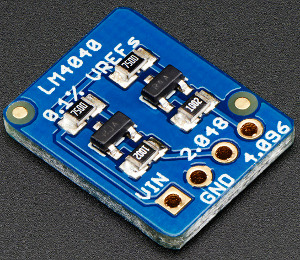If you connect that to AREF then you free yourself from the worry of wondering exactly what the reference voltage is. Is it 4.9V? 5V? 5.1V?

Also see below for a discussion about the TL431 voltage reference IC.

The library function analogRead "blocks", that is, it waits until the conversion is done. However you don't have to do that. This simple code below starts a conversion, and then checks in the main loop if it is finished. When it is finished it displays the result:

``````
const byte adcPin = 0;  // A0

bool working;

void setup ()
{
Serial.begin (115200);
Serial.println ();

}  // end of setup

void loop ()
{
if (!working)
{
working = true;
}

// the ADC clears the bit when done
{
working = false;
Serial.println (value);
delay (500);
}

}  // end of loop
``````

That lets you do other things (perhaps send a previous value to a PC) while taking another reading.

Read with an interrupt when done

This example code (from http://www.gammon.com.au/interrupts) shows reading asynchronously (non-blocking) and using the ADC complete interrupt to fetch the results.

``````

void setup ()
{
Serial.begin (115200);
ADMUX  =  bit (REFS0) | (adcPin & 0x07);    // AVcc and select input port

}  // end of setup

{

void loop ()
{
// if last reading finished, process it
{

// do something with the reading, for example, print it
delay (500);

}

// if we aren't taking a reading, start a new one
{
// start the conversion
}

// do other stuff here

}  // end of loop
``````

The ADC can only do one conversion at time but has a multiplexer that lets it choose from different inputs. On a Uno those inputs are A0 to A5, and on some boards (such as the Arduino Mini) you also can choose A6 and A7.

These are just presented as the low-order bits to the ADMUX register (A0 is 0, A1 is 1 and so on) like this:

``````
ADMUX = bit (REFS0) | (adcPin & 7);   // Use AVcc for conversion
``````

Tip:

The Arduino IDE defines A0 as 14 on the Uno, so that you can do a digitalWrite to A0 and not have it be confused with digital pin 0. When using analogRead the code subtracts 14 so that A0 becomes 0.

If you are using the suggested code here write "0" for analog input zero, not "A0".

Temperature sensor

Multiplexer input 0x08 is connected as an internal temperature sensor. The datasheet advises you to use that with the 1.1V reference voltage, like this:

``````
ADMUX = bit (REFS0) | bit (REFS1)  | 0x08;    // temperature sensor
``````

The datasheet suggest that a voltage thus obtained would be around 314 mV for a temperature of 25°C, however I personally found that the reading was somewhat higher than that. I got a reading of 337 which is equivalent to 362 mV.

``````
337.5 * 1.1 / 1024 * 1000 = 362.5  mV
``````

Still, they do suggest that each chip is different and that you should calibrate your own chip by measuring empirically and storing a suitable subtraction factor in EEPROM.

Example code that gave a reasonable result:

``````
void setup ()
{
Serial.begin (115200);
ADMUX = bit (REFS0) | bit (REFS1)  | 0x08;    // temperature sensor

delay (20);  // let it stabilize

{ }

Serial.print ("Temperature = ");
Serial.println (value - 320);
}  // end of setup

void loop () { }
``````

Internal voltage sensor

Another multiplexer input is the internal bandgap voltage.

This means that we are using the 1.1V reference as an input to the ADC. Since this will have a known value (1.1V) then if we compare that to AVCC then the lower that AVCC is, the higher a reading will be returned.

For example:

``````
1.1 / 5 * 1024 = 225
1.1 / 3.3 * 1024 = 341

In general:

1.1 / voltage * 1024.0 = reading

thus:

voltage = 1.1 / reading * 1024.0
``````

Example code:

``````
const float InternalReferenceVoltage = 1.096; // as measured

void setup ()
{
Serial.begin (115200);
ADMUX = bit (REFS0) | bit (MUX3) | bit (MUX2) | bit (MUX1);

delay (10);  // let it stabilize

{ }

float results = InternalReferenceVoltage / float (ADC + 0.5) * 1024.0;
Serial.print ("Voltage = ");
Serial.println (results);
}  // end of setup

void loop () { }
``````

For greater accuracy measure the actual 1.1V reference voltage (there is a sketch further up this page that will do that) and use that instead of hard-coding 1.1V.

The usefulness of this is that code can self-detect its own supply voltage. In particular, if you are connected to a battery, you could work out when the battery is reaching the end of its life, by using the above code.

Tip:

According to the datasheet:

Quote:

When the bandgap reference voltage is used as input to the ADC, it will take a certain time for the voltage to stabilize. If not stabilized, the first value read after the first conversion may be wrong.

Thus, if you are attempting to find AVCC using this technique, take two readings, ignoring the first one while the voltage stabilizes.

Measuring resistance

Quite a few sensors output a resistance, for example some temperature sensors, light-dependent resistors (LDRs), pressure-sensitive mats, and so on. To convert a resistance to a voltage we need a voltage-divider, like this: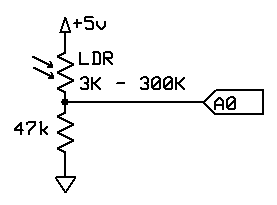The LDR resistance decreases as light increases, so the voltage divider there will have a higher voltage, the brighter the light is.

Measuring higher voltages

Sometimes you might want to check the voltage on a 12V or similar battery. You can't just connect that into the analog input or you will damage your Arduino. A voltage divider like this will help drop the voltage down: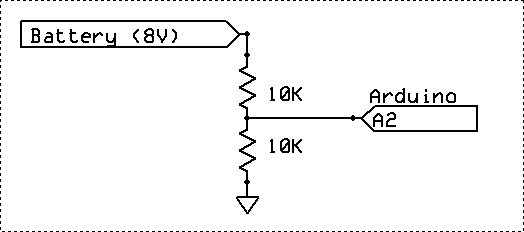In that case the analog pin would read 4V if the battery was 8V.

``````
10k / (10k + 10k) * 8 = 4V
``````

That circuit is OK for an 8V battery, but for a 12V battery the top 10k resistor would need to be bigger (say, 22k).

In that case we would get 3.75 on the analog pin if the battery was 12V.

``````
10k / (10k + 22k) * 12 = 3.75V
``````

- Nick Gammon

www.gammon.com.au, www.mushclient.com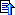top

Posted by Nick Gammon   Australia  (22,921 posts)bio   Forum Administrator
Date Reply #1 on Fri 27 Feb 2015 08:25 AM (UTC)

Amended on Sat 28 Feb 2015 02:56 AM (UTC) by Nick Gammon

Message
 How to interpret the results So how do we convert the result from analogRead to a voltage? Let's suppose: `````` int value = analogRead (A0); `````` And imagine we get the value of 1000. Now (ignoring the fact that integers don't have decimal places) is the voltage (assuming we have a 5V reference): `````` 1000.0 / 1024 * 5.0 = 4.8828125 or: 1000.0 / 1023 * 5.0 = 4.8875855 `````` In other words, divide by 1023 or 1024? I should point out that dividing by 1024 immediately gives a "wrong" answer for measuring 5V: `````` 1023.0 / 1024 * 5.0 = 4.9951171 `````` This is because the maximum reading is 1023. Browsing the web: http://arduino.cc/en/Tutorial/ReadAnalogVoltage Quote: To scale the numbers between 0.0 and 5.0, divide 5.0 by 1023.0 and multiply that by sensorValue One vote for 1023. http://forum.arduino.cc/index.php?topic=200447.0 Multiple schools of thought, including: Quote: You divide by 1024 if you are a mathematician or if you truly understand ADC successive approximation. http://blog.codeblack.nl/post/NetDuino-Getting-Started-with-ADC.aspx Hedging his bets: Quote: The number 0 represents 0V and 1023 represents Aref. The voltage level at any ADC port can be determined as follows: ``float analogValue = (float)digitalValue * (3.3f/ 1023f)`` In theory, you should divide by 1024, because there are that many steps. http://forums.netduino.com/index.php?/topic/2216-adc-value-divide-by-1024-or-1023/ Different opinions: Quote: The ADC returns a value from 0 to 1023 not 0 to 1024. 0 to 1023 is 1024 different values because zero is a value too. ... That is why you divide by 1023 and not 1024. Quote: Not exactly. Consider the nominal "width" of the ADC reading: it is 1024. That is strictly related to Vref (typically 3.3V). Now we must slice that segment in many smaller parts: in total are 1024 "tiles" (if you like numbered from 0 to 1023). http://forum.arduino.cc/index.php?topic=252276.0 Quote: The divisor (1023.0) in that tutorial is wrong. The correct value is 1024.0... ``float voltage= sensorValue * (5.0 / 1024.0);`` The maximum value returned by the analog-to-digital converter (1023) is correct. http://www.electro-tech-online.com/threads/why-adc-1024-is-correct-and-adc-1023-is-just-plain-wrong.132570/ Quote: The correct scaling math; *x/n or *x/1024 correctly scales all the output data in size, but gives an average rounding error of 0.5 ADC counts (always rounding down). So, how come we get a "wrong" result for a value of 1023? It seems that the ADC can return 1024 "slots" of values (from 0 to 1023) where each slot represents 1/1024 of the reference voltage. So for a 5V reference voltage the slot width is: `````` 5 / 1024 = 0.0048828125V `````` Thus a result of 0 could be: 0V to 0.00488V A result of 1 could be: 0.00488V to 0.009766V ... A result of 1023 could be: 4.99511V to 5V So really the "wrong" result for 5V is more that the voltage is not necessarily 5V, it could be 4.995. In addition to that, the hardware rounds down the result, so you could compensate by adding in an average error of 0.5. `````` 1023.5 / 1024 * 5.0 = 4.99756V `````` So this is reasonably close to 5V. Similarly a reading of 0 should probably be treated as possibly halfway between 0 and 1, thus it could on average be: `````` 0.5 / 1024 * 5.0 = 0.002441V `````` Oh yes, and the Atmega328 datasheet mentions in the section "23.7 ADC Conversion Result" that the figure is 1024. So how do we interpret the results, then? First, let's go back to the datasheet: Quote: 0x000 represents analog ground, and 0x3FF represents the selected reference voltage minus one LSB. So (contrary to what you might expect) 0x3FF (ie. 1023) does not claim to represent Vref, but rather the reference voltage minus one bit (which in the case of 5V is 5/1024 = 4.88 mV). Thus, the fact that dividing 1023/1024 and multiplying by 5 does not give 5 is entirely consistent with the fact that it does not claim to represent Vref. The datasheet goes on (on the previous page): Quote: Quantization Error: Due to the quantization of the input voltage into a finite number of codes, a range of input voltages (1 LSB wide) will code to the same value. Always ±0.5 LSB. So there is an expected error of +/- 2.44 mV. Therefore I suggest the formula: `````` float voltage = ((float) rawADC + 0.5 ) / 1024.0 * Vref; `````` Automatic mode You can get the ADC to trigger automatically, so it collects samples when some external event arrives, for example, a timer interrupt. This code demonstrates that: `````` const byte adcPin = 0; // A0 const int MAX_RESULTS = 256; volatile int results [MAX_RESULTS]; volatile int resultNumber; // ADC complete ISR ISR (ADC_vect) { if (resultNumber >= MAX_RESULTS) ADCSRA = 0; // turn off ADC else results [resultNumber++] = ADC; } // end of ADC_vect EMPTY_INTERRUPT (TIMER1_COMPB_vect); void setup () { Serial.begin (115200); Serial.println (); // reset Timer 1 TCCR1A = 0; TCCR1B = 0; TCNT1 = 0; TCCR1B = bit (CS11) | bit (WGM12); // CTC, prescaler of 8 TIMSK1 = bit (OCIE1B); // WTF? OCR1A = 39; OCR1B = 39; // 20 uS - sampling frequency 50 kHz ADCSRA = bit (ADEN) | bit (ADIE) | bit (ADIF); // turn ADC on, want interrupt on completion ADCSRA |= bit (ADPS2); // Prescaler of 16 ADMUX = bit (REFS0) | (adcPin & 7); ADCSRB = bit (ADTS0) | bit (ADTS2); // Timer/Counter1 Compare Match B ADCSRA |= bit (ADATE); // turn on automatic triggering // wait for buffer to fill while (resultNumber < MAX_RESULTS) { } for (int i = 0; i < MAX_RESULTS; i++) Serial.println (results [i]); } // end of setup void loop () { } `````` Timer 1 is generating interrupts at 50 kHz: `````` 1 / (62.5e-9 * 8 * 40) = 50000 Hz `````` I am feeding a sine wave at 4 kHz into A0, with these results: `````` 378 710 899 992 966 827 609 367 159 38 35 147 350 593 817 965 1001 917 733 494 259 88 21 76 239 471 711 905 1001 977 839 620 376 164 39 `````` It doesn't look exciting, until you graph it: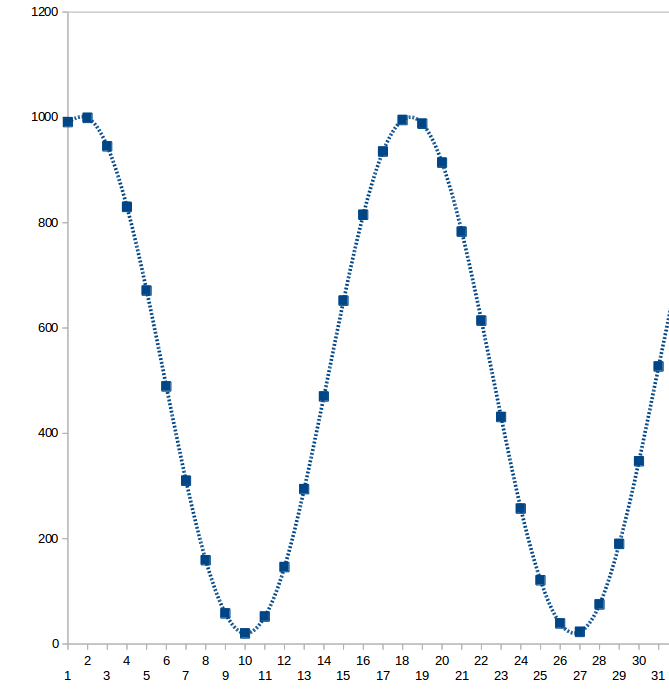Now a square wave: `````` 993 1003 1005 1006 1007 1008 1008 1008 1008 24 21 20 19 19 19 19 19 993 996 996 996 996 995 995 994 35 23 19 19 20 19 19 19 18 995 ``````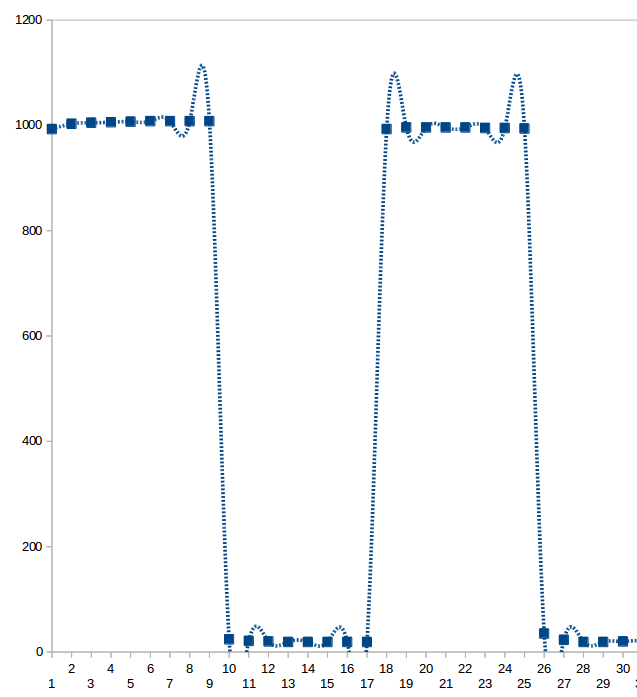Or a sawtooth wave: `````` 859 790 671 555 438 319 199 83 76 193 312 430 547 664 782 899 984 867 749 631 513 396 279 162 44 112 228 345 463 579 697 816 934 947 831 ``````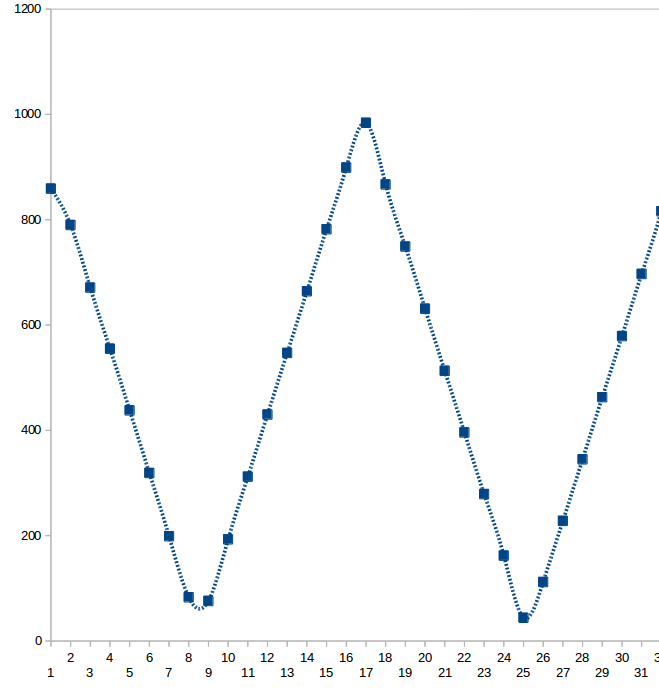This shows how you can use the ADC as a nice little (low frequency) oscilloscope. - Nick Gammon www.gammon.com.au, www.mushclient.comtop

Posted by Nick Gammon   Australia  (22,921 posts)bio   Forum Administrator
Date Reply #2 on Fri 27 Feb 2015 08:19 PM (UTC)

Amended on Sat 28 Feb 2015 03:11 AM (UTC) by Nick Gammon

Message
 Sleep during reading Another useful technique is to use the SLEEP_MODE_ADC (ADC sleep mode) while taking a reading. This lowers the processor's activity, reducing noise in the ADC circuitry. It also reduces power consumption which can be handy if you have a battery-powered device and want to keep the power consumed, while you are reading the ADC, to a lower level. `````` #include // Sleep Modes // when ADC completed, take an interrupt EMPTY_INTERRUPT (ADC_vect); // Take an ADC reading in sleep mode (ADC) float getReading (const byte port) { ADCSRA = bit (ADEN) | bit (ADIF); // enable ADC, turn off any pending interrupt ADCSRA |= bit (ADPS0) | bit (ADPS1) | bit (ADPS2); // prescaler of 128 ADMUX = bit (REFS0) | (port & 0x07); // AVcc noInterrupts (); set_sleep_mode (SLEEP_MODE_ADC); // sleep during sample sleep_enable(); // start the conversion ADCSRA |= bit (ADSC) | bit (ADIE); interrupts (); sleep_cpu (); sleep_disable (); // awake again, reading should be done, but better make sure // maybe the timer interrupt fired while (bit_is_set (ADCSRA, ADSC)) { } return ADC; } // end of getReading void setup () { int reading = getReading (0); Serial.begin (115200); Serial.println (); Serial.println (reading); } // end of setup void loop () { } `````` Digital input disable If you are doing only ADC conversions on some or all of the analog inputs, you should disable the digital buffers, to save power, like this: `````` bitSet (DIDR0, ADC0D); // disable digital buffer on A0 bitSet (DIDR0, ADC1D); // disable digital buffer on A1 bitSet (DIDR0, ADC2D); // disable digital buffer on A2 bitSet (DIDR0, ADC3D); // disable digital buffer on A3 bitSet (DIDR0, ADC4D); // disable digital buffer on A4 bitSet (DIDR0, ADC5D); // disable digital buffer on A5 `````` Once disabled, a digitalRead on those pins will always read zero. Alternatives You can get dedicated ADC chips (for example: MCP3201/2/4/8 with from 1 to 8 channels, and MCP3304) that interface by SPI. These can run alongside the Arduino, converting much faster than the inbuilt ADC, and have higher resolutions (eg. 12 to 14 bits). Analog comparator If you just want to test a single threshold (eg. are the lights on or off?) then the analog comparator may do the job and is much faster. Details here: http://www.gammon.com.au/forum/?id=11916 - Nick Gammon www.gammon.com.au, www.mushclient.comtop

Posted by Nick Gammon   Australia  (22,921 posts)bio   Forum Administrator
Date Reply #3 on Sat 28 Feb 2015 11:38 PM (UTC)

Amended on Sun 01 Mar 2015 09:53 AM (UTC) by Nick Gammon

Message
 TL431 voltage reference Whilst working on the above thread, I started exploring using the TL431 voltage reference IC. It is, apparently, very commonly used. For example it is described in this blog: Reverse-engineering the TL431: the most common chip you've never heard of. It is widely used as a voltage reference in power supplies, computers, LED drivers and so on. You can get 50 of them from eBay for \$US 1.60. I found the information in the datasheet a bit hard to follow, so here are my notes on using it. Wire it up the right way! It is easy to get the anode and cathode confused. Some datasheets list the pin order from the top, others from the bottom. Also the anode (positive side) goes to ground.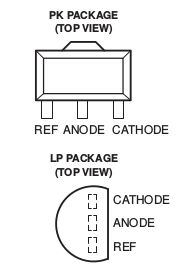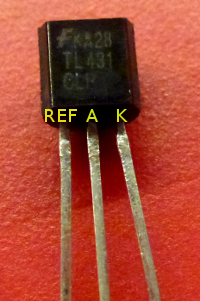Make a 2.5V reference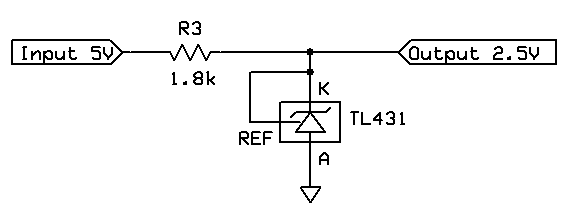The TL431 has an inbuilt "bandgap" reference, which provides a stable reference voltage. Wikipedia: Bandgap voltage reference The chip is designed to sink current from Cathode to Anode until the voltage at the reference pin is the reference voltage (2.5V). Thus, if VREF is higher than 2.5V the chip conducts, sinking current, until VREF is back at 2.5V. The simple use then, is to make a 2.5V reference, as in the schematic above. But, what value to use for R3? (I call it R3 because another couple of resistors, to be explained shortly, are called R1 and R2 in the datasheet). (I'm genuinely interested to know why, in a datasheet, they label two resistors R1 and R2, but leave the third one unlabelled. Is it the "unimportant resistor"?). We know these things: The voltage across R3 must be 2.5V (in this case) because I have a 5V supply. Assuming the current drawn by my load (Output) is negligible, the chip must sink enough current to cause that voltage drop over R3. There is a minimum amount of current needed for the voltage reference to work. From the datasheet: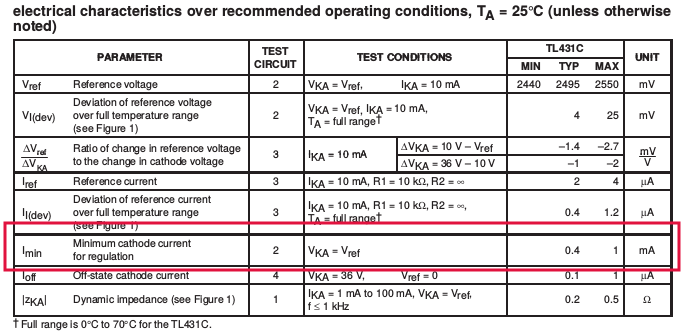That suggests that a minimum of 0.4 mA is needed, but 1 mA is the "maximum minimum". There is a maximum amount of current that it will sink. From the datasheet: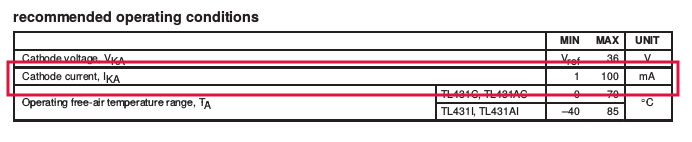Thus we don't want to sink more than 100 mA. The recommended operating conditions are to sink from 1 to 100 mA. Given that, let's work out what resistance we need for this particular voltage drop using Ohm's Law: `````` R = V / I R = 2.5 / 0.001 R = 2.5k `````` The nearest standard value is 2.2k, which gives us this current consumption: `````` I = V / R I = 2.5 / 2200 I = 0.001136 (that is 1.136 mA) `````` Thus for this circuit, a 2.2k resistor lets us operate within parameters. Wiring it up confirms that it works as expected. However, see below for choosing a different resistor (1.8k) to allow for a range of input voltages. Make a 4V reference The TL431 can provide other reference voltages by using a voltage divider (resistors R1 and R2) at the REF pin. The objective here is that the voltage divider divides down the voltage at the Cathode so that, if the desired voltage is at the Cathode, then 2.5V will be at the REF pin.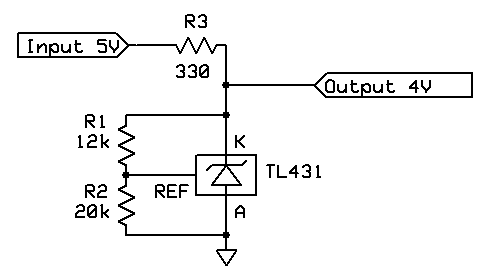This is how we do that ... We can use a Voltage Divider Calculator to simplify our work. The datasheet hints that 10k is a reasonable value for R1, so let's start with that.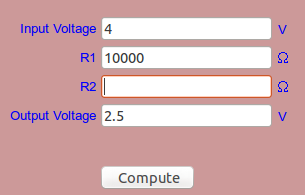Hitting "Compute" gives us 16.666k for R2, which is not a particularly easy value to work with. `````` R2 = R1 * (1 / (Vin / Vout - 1)) R2 = 10000 * (1 / (4 / 2.5 - 1)) R2 = = 16666.67 `````` As a guess, let's try 12k for R1 and see where that leads us: `````` R2 = R1 * (1 / (Vin / Vout - 1)) R2 = 12000 * (1 / (4 / 2.5 - 1)) R2 = 20000 `````` That's better! A 12k resistor is a standard value, and we can make up 20k by putting 2 x 10k in series. Now we need to calculate R3, such that over 1 mA flows through the device, but less than 100 mA. First let's work out the current flow through R1 and R2. `````` R1 + R2 = 12000 + 20000 = 32000 I = V / R I = 4 / 32000 I = 0.000125 (0.125 mA) `````` That means our target current through R3 is going to be 1.125 mA or more. (1 mA for the chip and 0.125 mA for R1 and R2 in series). We now only have a 1V voltage drop over R3, so we need a suitable resistor, such that we get 1.125 mA from a 1V voltage drop. `````` R = V / I R = 1 / 0.001125 R3 = 888 `````` Since we want more, rather than less, current, let's go for the next standard resistor size down, namely 560 ohms. `````` I = V / R I = 1 / 560 I = 0.001786 (1.786 mA) `````` This seems to be acceptable. We now have values for R1, R2 and R3. Measurement confirms that in my test case, the output is 4.017V and the current consumption is 1.763 mA. Tip: The measured results seems little high, after all we were aiming for 4.000V and this is a precision voltage reference, right? The answer lies in the datasheet. `````` VKA = Vref * (1 + R1/R2) + Iref * R1 `````` The (small) amount of current flowing into the REF pin (Iref) influences the final voltage. According to the datasheet it is around 2 µA, so we need to multiply that by R1 (12k), giving this result: `````` 2.5 * (1 + 12000/20000) + 2E-6 * 12000 = 4.024V `````` So we are really expecting 4.024V on the cathode, not 4.000V exactly. We didn't get precisely 4.024V either, but (apart from probable slight errors in resistor values) the reference voltage is closer to 2.495V in this particular chip. Substituting that more accurate figure instead of 2.5V gives us: `````` 2.495 * (1 + 12000/20000) + 2E-6 * 12000 = 4.016 `````` So the output was very close to the theoretical output. Different supply voltages The calculations above assume that the supply (input) voltage is 5V. But what if it varies from 4.5V to 5.5V? We need to ensure that the chip remains in spec. Let's rework for different input voltage ... 2.5V reference - 4.5V supply `````` Voltage drop over R3 = 4.5 - 2.5 = 2V R3 = 2 / 0.001 R3 = 2000 (2k) `````` 2.5V reference - 5.5V supply `````` Voltage drop over R3 = 5.5 - 2.5 = 3V R3 = 3 / 0.001 R3 = 3000 (3k) `````` Summary for 2.5V reference So a resistor in the range 2k to 3k is required to handle that range of input voltages. Let's use the next lowest standard value: 1.5k. `````` For 5.5V: I = 3 / 1500 = 2 mA For 4.5V: I = 2 / 1500 = 1.33 mA `````` Thus the 1.5k resistor will keep us in the operating range of 1 mA to 100 mA for input voltages of 4.5V to 5.5V. 4V reference - 4.5V supply `````` Voltage drop over R3 = 4.5 - 4 = 0.5V R3 = 0.5 / 0.001125 R3 = 444 ohms `````` 4V reference - 5.5V supply `````` Voltage drop over R3 = 5.5 - 4 = 1.5V R3 = 1.5 / 0.001125 R3 = 1333 (1.3k) `````` Summary for 4V reference So a resistor in the range 444 to 1333 is required to handle that range of input voltages. Let's use the next lowest standard value: 330. `````` For 5.5V: I = 1.5 / 330 = 4.5 mA For 4.5V: I = 0.5 / 330 = 1.5 mA `````` Thus the 330 ohm resistor will keep us in the operating range of 1 mA to 100 mA for input voltages of 4.5V to 5.5V. Accuracy The datasheet quotes 30 ppm/°C, which means the output should vary by 30 µV per degree. - Nick Gammon www.gammon.com.au, www.mushclient.comtop

Posted by Nick Gammon   Australia  (22,921 posts)bio   Forum Administrator
Date Reply #4 on Sun 01 Mar 2015 10:27 PM (UTC)
Message
 TL431 current reference While we are on the subject of references, after watching a video by Martin Lorton I made up a current reference as well: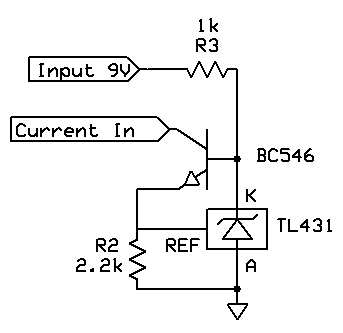The theory here is that R2 is a current sense resistor, and you can adjust it to give a desired current sink from the "Current In" pin, according to this formula: `````` Current = 2.5 / R2 `````` In my case I used 2.2k for R2, so the expected current sink was: `````` 2.5 / 2200 = 0.001136 (1.136 mA) `````` Tests showed that this was pretty close. The idea is that the TL431 adjusts the current on the transistor base in such a way that 2.5V is on the reference pin. Since we know the voltage drop (2.5V) and the resistance (2.2k) therefore we know what the current through that resistor must be to achieve that. - Nick Gammon www.gammon.com.au, www.mushclient.comtop

Posted by Nick Gammon   Australia  (22,921 posts)bio   Forum Administrator
Date Reply #5 on Sun 10 Apr 2016 04:16 AM (UTC)

Amended on Sun 10 Apr 2016 04:19 AM (UTC) by Nick Gammon

Message
 How does the ADC work? The ATmega328P features a 10-bit successive approximation ADC. The ADC is connected to an 8-channel Analog Multiplexer.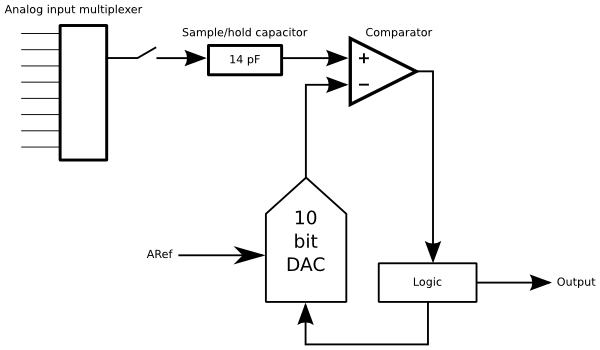First, an input is selected from the multiplexer on the left. This allows any of 8 input channels to be active at one time (but only one). These are: A0 to A5 on the Uno (some boards also have A6 and A7). There are also additional input channels (not shown) which also allows the selection of: The internal temperature sensor Ground Bandgap reference voltage The input channel (multiplexer output) is connected to a "sample and hold" capacitor, as shown. Once an analog conversion starts the switch is opened after 1.5 ADC cycles (12 µs with the default prescaler of 128) so that the capacitor retains its current reading (ie. it holds it) so that the input voltage can now change without affecting the result. This would be useful for fast-changing signals, as the whole conversion can take 104 µs (depending on the prescaler). To work out the voltage the logic applies the analog reference voltage (ARef) divided down by a DAC (digital-to-analog converter) under the control of the ADC logic. The output of the DAC is sent to the comparator which determines if the input voltage is higher or lower than the reference voltage, at its current divided state. For example, the high-order bit would be set or cleared by setting the DAC to divide the reference voltage by 2 (ie. 2.5V if ARef is 5V). If the input is higher than 2.5V then the high-order bit in the result is set. This takes one ADC cycle (8 µs at the default prescaler of 128 with a 16 MHz processor clock). To get the next result bit the DAC would be set to the next step (ie. 0.25 of ARef or 0.75 of ARef depending on whether the first bit was set or not) and another comparison done. This process is repeated for all 10 bits. Sample-and-hold capacitor timing The time taken to charge the sample-and-hold capacitor will be affected by two things: The frequency of the input signal The impedance of the signal A capacitor is reckoned as having fully charged (or fully discharged depending on which way you are going) in 5τ (where τ is R*C), so you could therefore calculate that for a given resistor and capacitor it will take: `````` 5 * R * C `````` In fact it will charge to 99.33% in that time, where 99.33% comes from: `````` 1 - e-5 = 0.9933 `````` The input impedance is given in the datasheet as being from 1 to 100k (depending on the frequency), and they recommend a source signal impedance of 10k. Knowing that, and the value of the 14 pF capacitor, we can see that it will charge in: `````` 5 * 110e3 * 14e-12 * 1e6 = 7.7 µs `````` This means it should fully charge (or near enough) in the 12 µs that you are allocated from the start of the conversion to when the sample-and-hold capacitor is disconnected from the source. Conversion timing As mentioned earlier in this thread, you can speed up ADC conversions by using a smaller prescaler. This reduces the amount of time available to calculate each bit value. The smallest prescaler which gave reasonable results was 16, meaning that each ADC cycle was 1 µs. Time is required for: The DAC output to settle to the configured fraction of ARef The comparator to compare the DAC output to the sample-and-hold capacitor value Logic to make decisions To take 1 µs for all of the above sounds reasonable. - Nick Gammon www.gammon.com.au, www.mushclient.comtop

The dates and times for posts above are shown in Universal Co-ordinated Time (UTC).

To show them in your local time you can join the forum, and then set the 'time correction' field in your profile to the number of hours difference between your location and UTC time.

177,110 views.Refresh page

Go to topic:           Search the forumtop

Quick links: MUSHclient. MUSHclient help. Forum shortcuts. Posting templates. Lua modules. Lua documentation.

Information and images on this site are licensed under the Creative Commons Attribution 3.0 Australia License unless stated otherwise.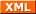Forum RSS feed ( https://gammon.com.au/rss/forum.xml )